Tutorial 15 – Write data into excel, Read from properties file

Welcome to the fifteenth tutorial of Selenium-Python series! In this tutorial, we will study about how to write data into excel & read data from properties file. Please go through the previous article before going through this one.

What you will Learn:
Write by column index
Write by column name
Property file
Configparser
Create properties file
Read data in form of dictionary
Conclusion

Write by column index
We will continue using the same python module that we used in previous article. See figure 1. To write into the excel file using column index, we will create a function that will accept the sheetName, rowIndex, colIndex & the value that we would like to write into the cell (line 12)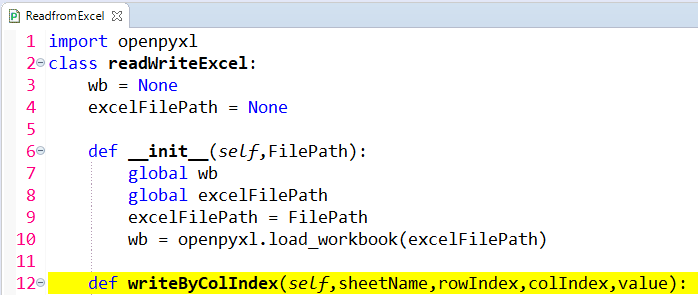Figure 1

Now see below line number 14. This will write the value to whatsoever rowIndex & colIndex we will give.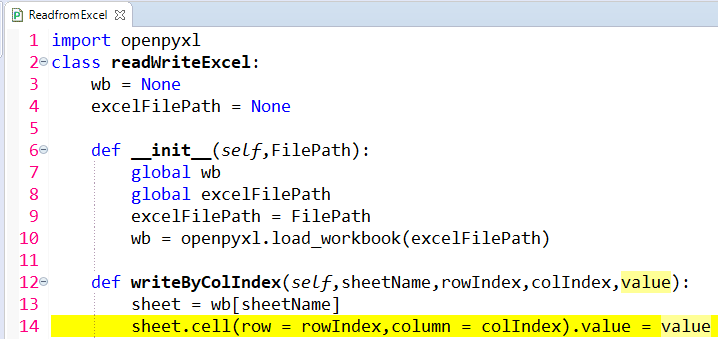Figure 2

So whatsoever we will write to a particular cell, we have to save that to a workbook. For that, see line 15 below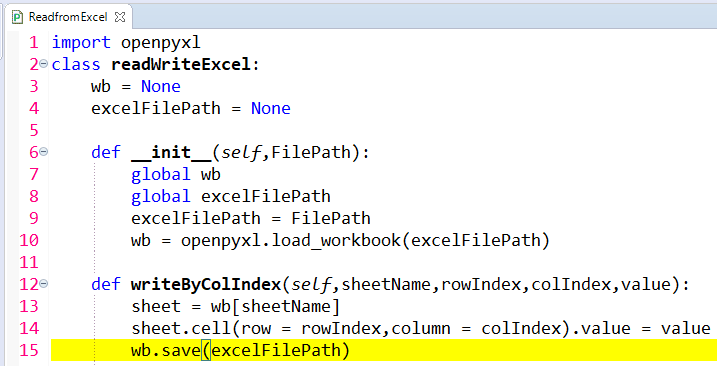Figure 3

We will call this function in ‘readwrite.py’ module, see line 5 below.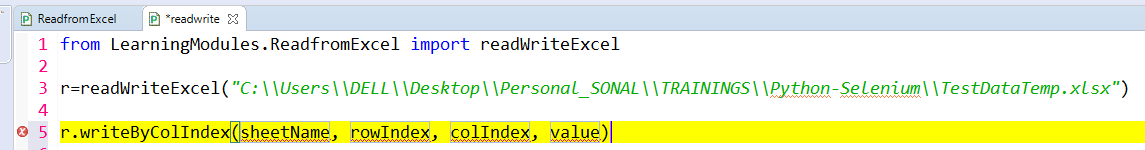Figure 4

Now see below figure. Suppose we want to write ‘Canada’ in rowIndex 4 and colIndex 3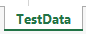Figure 5

See below, mention the sheetname, row index, column index & value parameters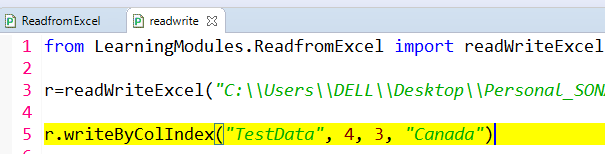Figure 6

Close the excel.

Run program. Notice below that ‘Canada’ is now written in place of ‘China’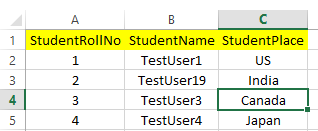Figure 7

Close the excel.

Write by column name
See below, we create a function to write by column name.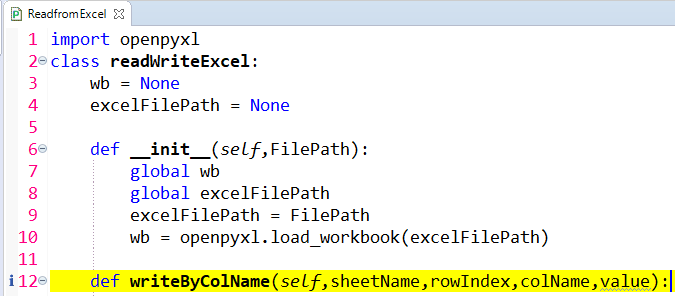Figure 8

See line 14 below. Let us initialize column index to 1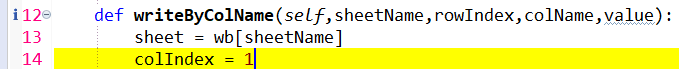Figure 9

Now we will write a ‘while’ loop.
We will check if the value of row number=1 & column number = ‘colIndex’ (that we already initialized to 1) is NOT equal to blank, see line 15Figure 10

We will than use the ‘if’ statement (line 16). What it means is that, if the colName (that we pass when we call this function) is equal to the cell value, than it will simply break (line 17)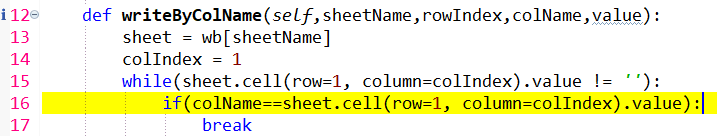Figure 11

If the colName (that we pass when we call this function) is NOT equal to the cell value, than it will increase the colIndex by 1 (line 18). After increasing the colIndex, we will again check using the while loop if the colName (that we pass when we call this function) is equal to the cell value, than it will break, otherwise again the colIndex will be incremented by 1 & process repeats.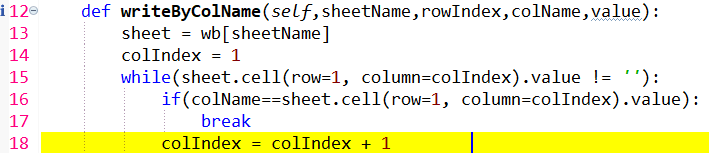Figure 12

At the end, we will set the cell value, line 19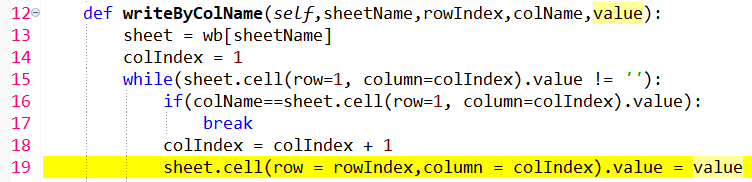Figure 13

Lastly we will save the file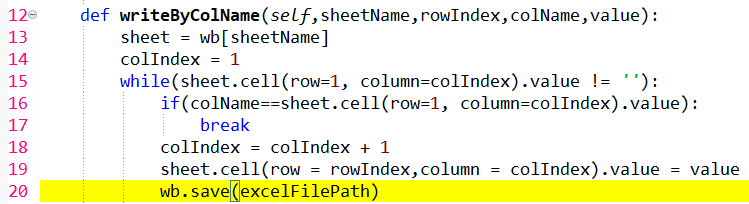Figure 14

Let us call this function, see below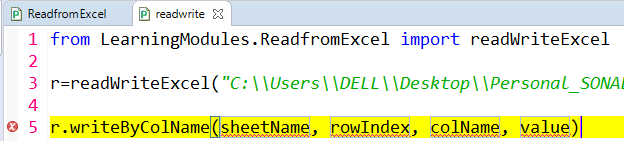Figure 15

Let us suppose we want to write ‘TestUser100’ in row index 5 & column name ‘StudentName’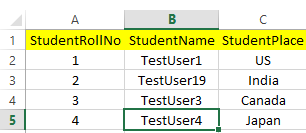Figure 16

So we have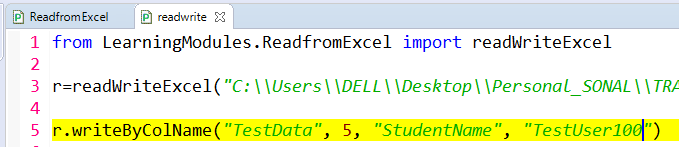Figure 17

Close excel

Run program, notice that ‘TestUser100’ is written in the cell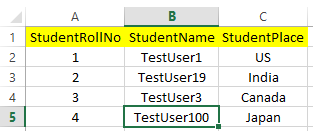Figure 18

Below is how the logic is working: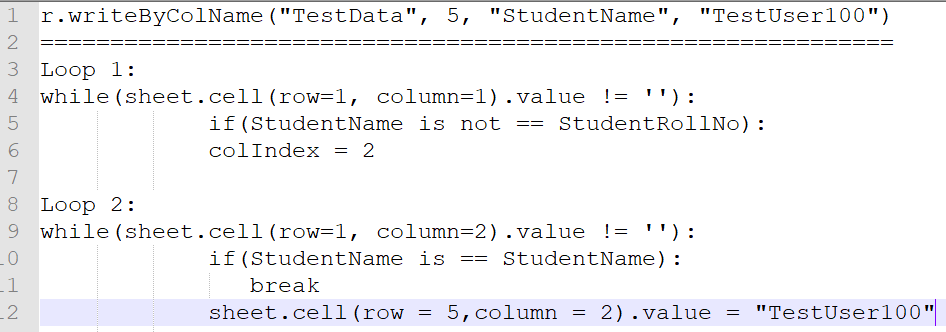Figure 19

Property file
In testing we have test cases that contain test data. There are 2 types of data:
a) Data that changes frequently (example, data such as username, password), this data is regular test data. To store this type of data, we use excel file.
b) Data that does not change frequently (example, url, page title, xpaths). To store this type of data, we use properties file. Thus the properties file has less changing data.

So let us say we have 3 web pages having same url & page title, see below. If we would like to change the url & page title of each of the 3 pages, we change them in the properties file instead of changing in all the individual pages. The data in the properties file is stored in the form of key=value pair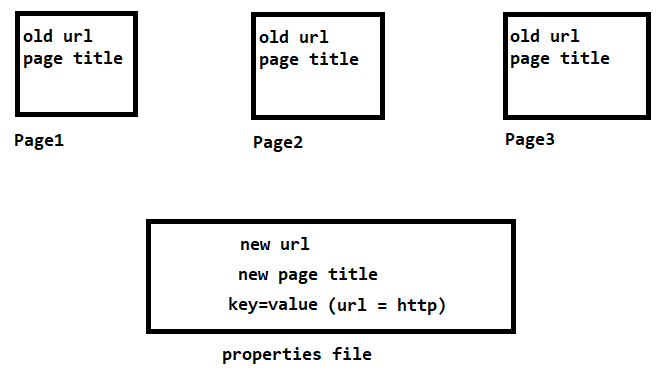Figure 20

Configparser
configparser is used to read the properties file. We will first install this configparser using command prompt, see below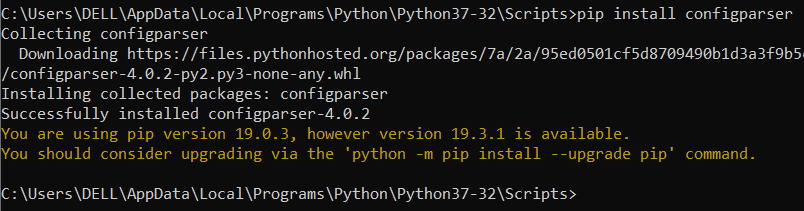Figure 21

Create properties file
To create a properties file, right click package > New >File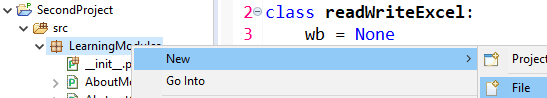Figure 22

Mention filename, see below. Instead of .properties, you can also use .ini extension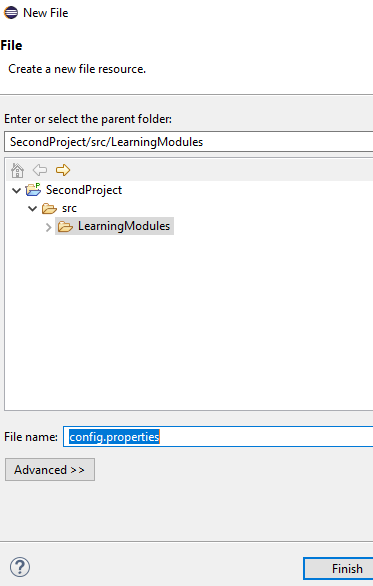Figure 23

Click Finish, the properties file gets created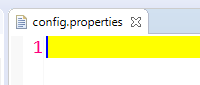Figure 24

Let us add some data to this file, so we have added 2 sections. The first section contains name/value pair & second section contains environment/value pair. So the data is in key/value pair form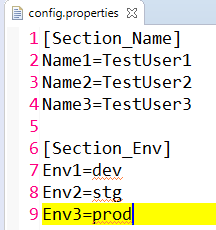Figure 25

Now we will read this properties file. To do that, let us create a new python module ‘PropertiesFile.py’. We will import the configparser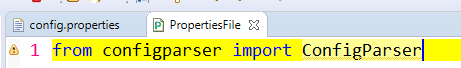Figure 26

To read the properties file, we write lines 2-5 seen below. In ine33 we are reading the properties file. In line#4 we are storing the sections in a variable.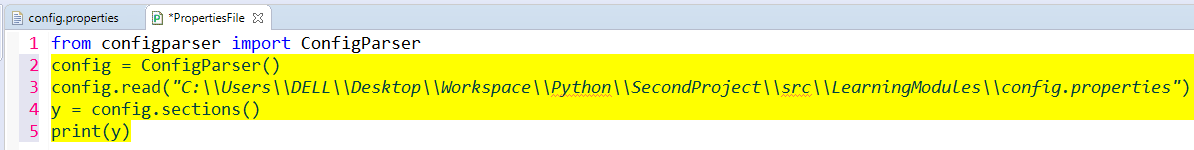Figure 27

So when we run this, the sections are printed, see below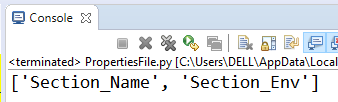Figure 28

To print the value of any name from the ‘Section_Name’, see line#7 below. This returns the value of ‘Name1’ key.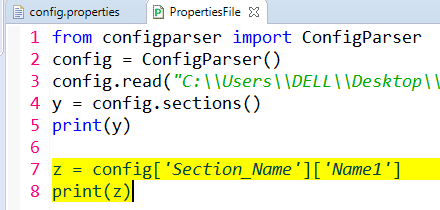Figure 29

When we run , we see ‘TestUser1’ gets printed in console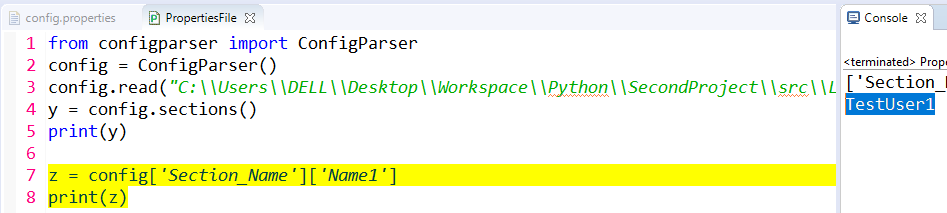Figure 30

Similarly you can fetch the values of other keys in the same or different sections, see below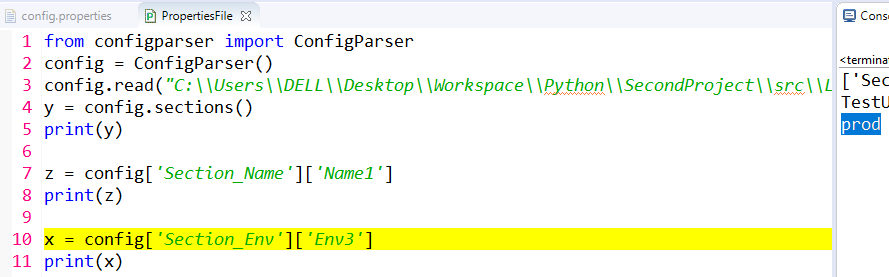Figure 31

Read data in form of dictionary
If you want to fetch data in the form of dictionary, we write lines 13-14 below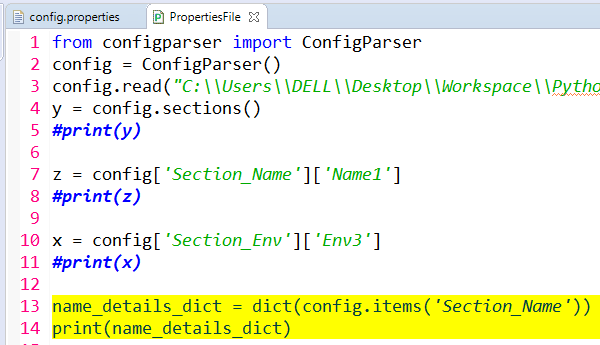Figure 32

When you run, the data gets printed in the form of dictionary, see below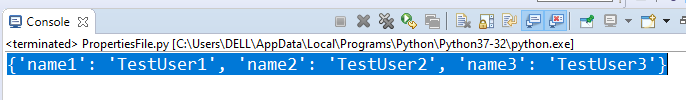Figure 33

Similarly we can fetch environments, see below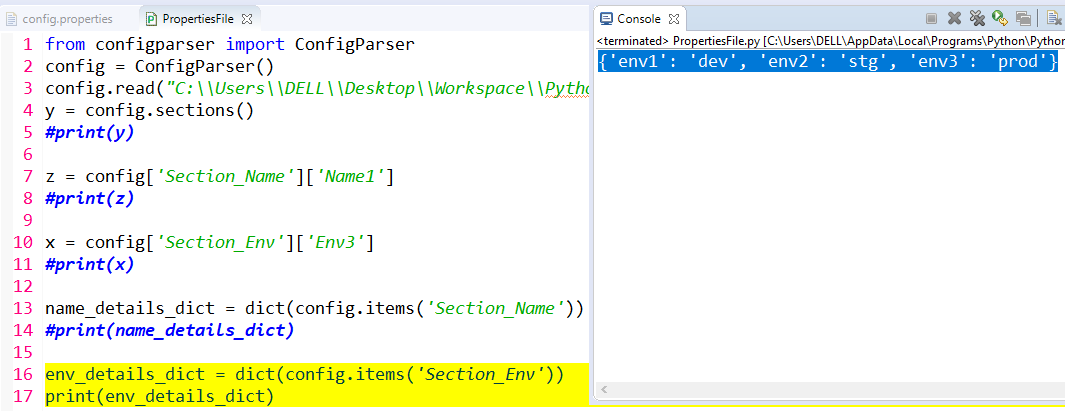Figure 34

Conclusion
In this tutorial we have studied about writing data into excel using openpyxl utility & reading data from properties file. Thank you!